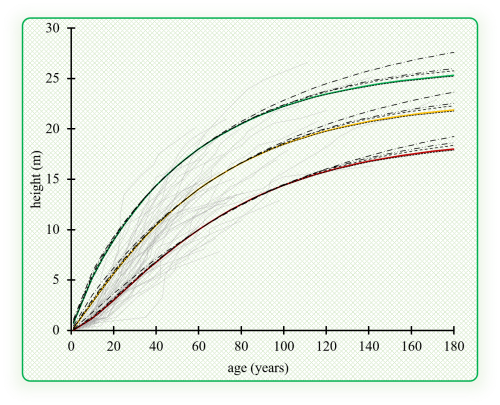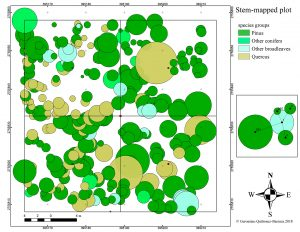# How to correct the heteroscedasticity and autocorrelation of residuals in taper and height growth models?Abstract

In modeling of taper functions and dominant height growth with time series data, the presence of heteroscedasticity and autocorrelation in residuals is common. Variance Functions (varFunc) and correlation structures (corStruct) were used to correct heteroscedasticity and autocorrelation; both were combined and evaluated through taper and height growth equations for Pinus teocote in Durango, Mexico. A dataset of 51 stems analysis with 768 taper observations and 634 height growth observations was used. The varFuncs applied were: 1) power function (varPower); 2) exponential function (varExp); 3) constant plus power function (varConstPower); and 4) a combination of power and exponential functions (varComb). The corStructs were: compound symmetry (corCompSymm), autoregressive of order 1 (corAR1), continuous-time autoregressive of order 1 (corCAR1), autoregressive-moving average (corARMA2-0), corARMA1-1, corARMA2-1, corARMA2-2, corARMA3-1 and corARMA3-2. To fit the equations, the generalized nonlinear least squares method was used and evaluated with a rating system through: RMSE, R^2, AIC, BIC, LogLik, VC and average bias. According to the rating system, the best combinations for taper and height growth equations were 1-9, 2-5, 3-8 and 4-6 and 1-6, 2-9, 3-7 and 4-4,respectively. In the taper equation, only the combination 2-5 was homoscedastic with independent residuals, and the selected height growth equations were homoscedastic with independent residuals; the varFunc and corStruct had influence on the trajectories of site index curves.

# Considering neighborhood effects improves individual dbh growth models for natural mixed-species forests in Mexico22